×#### Thank you for registering.

One of our academic counsellors will contact you within 1 working day.

Click to Chat

1800-1023-196

+91-120-4616500

CART 0

• 0

MY CART (5)

Use Coupon: CART20 and get 20% off on all online Study Material

ITEM
DETAILS
MRP
DISCOUNT
FINAL PRICE
Total Price: Rs.

There are no items in this cart.
Continue Shopping• Complete JEE Main/Advanced Course and Test Series
• OFFERED PRICE: Rs. 15,900
• View Details

```Chapter 20: Mensuration Exercise 20.4

Question: 1

Question 1. Find the area of a rectangle, whose

(i) Length = 6cm, breadth = 3 cm

(ii) Length = 8 cm, breadth = 3 cm

(iii) Length = 4.5 cm, breadth = 2 cm

Solution:

(i) Area of a rectangle = Length × Breadth

Length = 6 cm Breadth = 3 cm

Area of rectangle = 6 × 3 = 18 cm2

(ii) Area of a rectangle = Length × Breadth

Length = 8 cm Breadth = 3 cm

Area of rectangle = 8 × 3 = 24 cm2

(iii) Area of a rectangle = Length × Breadth

Length = 4.5 cm

Area of rectangle = 4.5 × 2 = 9 cm2

Question: 2

Find the area of a square whose side is:

(i) 5 cm

(ii) 4.1 cm

(iii) 5.5 cm

(iv) 2.6 cm

Solution:

Area of a square = Side × Side

(i) Side of the square = 5 cm

Area of the square = 5 × 5 = 25 cm2

(ii) Side of the square = 4.1 cm

Area of the square = 4.1 × 4.1 = 16.81 cm2

(iii) Side of the square = 5.5 cm

Area of the square = 5.5 × 5.5 = 30.25 cm2

(iv) Side of the square = 2.6 cm

Area of the square = 2.6 × 2.6 = 6.76 cm2

Question: 3

The area of a rectangle is 49 cm2 and its breadth is 2.8 cm. Find the length of the rectangle.

Solution:

Area = 49 cm2 Breadth = 2.8 cm

Area of the rectangle = Length × Breadth

= 49/2.8 = 17.5 cm

Question: 4

The side of a square is 70 cm. Find its area and perimeter.

Solution:

Side of the square = 70 cm

Area of the square = Side × Side = 70 × 70 = 4900 cm2

Perimeter of the square = 4 × Side

= 4 × 70 = 280 cm

Question: 5

The area of a rectangle is 225 cm2 and its one side is 25 cm, find its other side.

Solution:

Area = 225 cm2

One of the sides = 25 cm

Area of the rectangle = Product of the lengths of its two side

Other side = Area/Side = 225/25 = 9 cm

Question: 6

What will happen to the area of a rectangle if its

(i) Length and breadth are trebled (ii) Length is doubled and breadth is same

(iii) Length is doubled

Solution:

(i) If the length and breadth of a rectangle are trebled.

Let the initial length and breadth be l and b, respectively.

Original area = l × b = lb

Now,

the length and breadth are trebled which means they become three times of their original value.

Therefore New length = 3l

New area = 3l × 3b = 9lb

Thus, the area of the rectangle will become 9 times that of its original area.

(ii) If the length is doubled and the breadth is same.

Let the initial length and breadth be l and b, respectively.

Original area = l × b = lb

Now, length is doubled and breadth remains same.

Therefore New length = 2l

New area = 2l × b = 2 lb

Thus, the area of the rectangle will become 2 times that of its original area.

(iii) If the Length is doubled and breadth is halved.

Let the initial length and breadth be land b, respectively.

Original area =l × b = lb

Now, length is doubled and breadth is halved.

Therefore New length = 2l

New area = 2 l × b / 2 = lb

New area is also lb.

This means that the areas remain the same.

Question: 7

What will happen to the area of a square if its side is :

(i) Tripled

(ii) increased by half of it

Solution:

(i) Let the original side of the square be s.

Original area = s x s = s2

If the side of a square is tripled, new side will be equal to 3s.

New area = 3s × 3s = 9s2

This means that the area becomes 9 times that of the original area.

(ii) Let the original side of the square be s.

Original area = s × s = s2

If the side of a square is increased by half of it, new side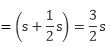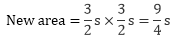This means that the area becomes 9/4 times that of the original area.

Question: 8

Find the perimeter of a rectangle whose area is 500 cm2 and breadth is 20 cm.

Solution:

Area = 500 cm2

Area of rectangle = Length × Breadth

= 500/20 = 25 cm

Perimeter of a rectangle = 2 (Length + Breadth)

= 2(25 + 20) cm = 2 × 45 cm = 90 cm

Question: 9

A rectangle has the area equal to that of a square of side 80 cm. If the breadth of the rectangle is 20 cm,  Find its length.

Solution:

Side of the square = 80 cm

Area of square = Side × Side = 80 × 80 = 6400 cm2

Given that:

Area of the rectangle = Area of the square = 6400 cm2

Breadth of the rectangle = 20 cm

Applying the formula:

Length of the rectangle = Area/Breadth

We get:

Length of the rectangle = 6400/20 = 320 cm

Question: 10

Area of a rectangle of breadth 17 cm is 340 cm2. Find the perimeter of the rectangle.

Solution:

Area of the rectangle = 340 cm2

Breadth of the rectangle = 17 cm

Applying the formula:

Length of a rectangle = Area / Breadth

We get:

Length of the rectangle = 340/17 = 20 cm

Perimeter of rectangle = 2 (Length + Breadth)

= 2 (20 + 17)

= 2 × 37

= 74 cm

Question: 11

A marble tile measures 15 cm × 20cm. How many tiles will be required to cover a wall of size 4m × 6m?

Solution:

Dimensions of the tile = 15 cm × 20 cm

Dimensions of the wall = 4 m × 6 m = 400 cm × 600 cm (Since, 1 m = 100 cm)

Area of the tile = 15 cm × 20 cm = 300 cm2

Area of the wall = 400 cm × 600 cm = 2, 40, 000 cm2Question: 12

A marble tile measures 10 cm × 12 cm. How many tiles will be required to cover a wall of size 3m × 4m? Also, find the total cost of the tiles at the rate of Rs 2 per tile.

Solution:

Dimension of the tile = 10 cm × 12 cm

Dimension of the wall =3 m × 4 m = 300 cm × 400 cm (Since, 1 m = 100 cm)

Area of the tile = 10 cm × 12 cm = 120 cm2

Area of the wall = 300 cm × 400 cm = 1, 20, 000 cm2

Number of tiles required to cover the wall = Area of wall / Area of one tile

= 120000/120 = 1,000 tiles

Cost of tiles at the rate of Rs. 2 per tile = 2 × 1,000 = Rs. 2,000

Question: 13

One tile of a square plot is 250 m, find the cost of leveling it at the rate of Rs 2 per square meter.

Solution:

Side of the square plot = 250 m

Area of the square plot = Side × Side = 250 × 250 = 62,500 m2

Rate of leveling the plot = Rs. 2 per m2

Cost of leveling the square plot = Rs. 62,500 × 2 = Rs. 1, 25, 000

Question: 14

The following figures have been split into rectangles. Find the areas. (The measures are given in centimeters)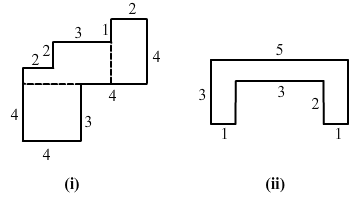Solution: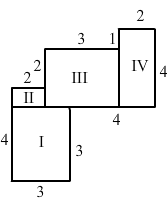(i) This figure consists of two rectangles II and IV and two squares I and III.

Area of square I = Side × Side = 3 × 3 = 9 cm=2

Similarity, area of rectangle II = (2 × 1) = 2 cm2

Area of square III = (3 × 3) = 9 cm2

Area of rectangle IV = (2 × 4) = 8 cm2

Thus, the total area of this figure = (Area of square I + Area of rectangle II + Area of square III + Area of rectangle IV) = 9 + 2 + 9 + 8 = 28 cm2

(ii) This figure consists of three rectangles I, II and Ill.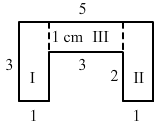Area of rectangle I = Length × Breadth = 3 × 1 = 3 cm2

Similarly, area of rectangle II = (3 × 1) = 3 cm2

Area of rectangle III = (3 × 1) = 3 cm2

Thus,

the total area of this figure = (Area of rectangle I + area of rectangle II + area of rectangle III)

= 3 + 3 + 3 = 9 cm2

Question: 15

Split the following shapes into rectangles and find the area of each. (The measures are given in centimeters)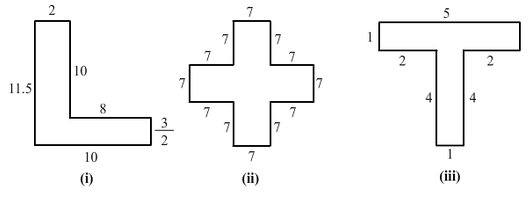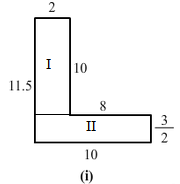Solution:

(i) This figure consists of two rectangles I and II.

The area of rectangle I = Length × Breadth = 10 × 2 = 20 cm2

Similarly, area of rectangle II = 10 × 32 = 15 cm2

Thus, total area of this figure = (Area of rectangle I + Area of rectangle II) = 20 + 15 = 35 cm2

(ii) This figure consists of two squares I and III and one rectangle II.

Area of square I = Area of square III = Side × Side = 7 × 7 = 49 cm2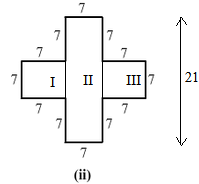Similarly, area of rectangle II = (21 × 7) = 147 cm2

Thus, total area of this figure = (Area of square I + Area of rectangle II + Area of square III)

= 49 + 49 + 147 = 245 cm2

(iii) This figure consists of two rectangles I and II.

Area of rectangle I = Length × Breadth = 5 × 1 = 5 cm2

Similarly, area of rectangle II = 4 × 1 = 4 cm2Thus, total area of this figure = (Area of rectangle I + Area of rectangle II) = 5 + 4 = 9 cm2

Question: 16

How many tiles with dimension 5 cm and 12 cm will be needed to fit a region whose length and breadth are respectively?

(i) 100 cm and 144 cm

(ii) 70 cm and 36 cm

Solution:

(i) Dimension of the tile = 5 cm × 12 cm

Dimension of the region = 100 cm × 144 cm

Area of the tile = 5 cm × 12 cm = 60 cm2

Area of the region = 100 cm × 144 cm = 14,400 cm2

Number of tiles required to cover the region = Area of the region / Area of one tile

= 14400/60 = 240 tiles

(ii) Dimension of the tile = 5 cm × 12 cm

Dimension of the region = 70 cm × 36 cm

Area of the tile = 5 cm × 12 cm = 60 cm2

Area of the region = 70 cm × 36 cm = 2,520 cm2

Number of tiles required to cover the region = Area of the region / Area of one tile

= 2520/42 = 42 tiles

```### Course Features

• 728 Video Lectures
• Revision Notes
• Previous Year Papers
• Mind Map
• Study Planner
• NCERT Solutions
• Discussion Forum
• Test paper with Video Solution